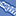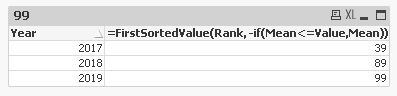# QlikView App Dev

Discussion Board for collaboration related to QlikView App Development.

Announcements
Action-Packed Learning Awaits! QlikWorld 2023. April 17 - 20 in Las Vegas: REGISTER NOW
cancel
Showing results for
Did you mean:Contributor

## How to use nested aggregation including set analysis - Little Tricky

Hi,

I have a table in excel. Please see the attachment. I need to create a simple straight table with the following requirement.

Year is the Only dimension.

The expression should take the Column "Value", compare it with the column "Mean" and display the Column "Rank". Final output is Year as dimension and Rank as Measure. Column "Value" changes for every year.

Example,  For Year 2017, the Column "Value" is 70. So, 70 should be compared against the column "Mean".  Once the Mean is greater than the Column "Value", then take the corresponding Rank. In this case, for Year 2017, the value 70 exceeds the mean at Rank 39. So for 2017 it should be displayed as 39.

Similarly, for Year 2018, the value 80 exceeds the mean at Rank 89. So for 2018 it should be displayed as 89.

Similarly, for Year 2019, the value 90 exceeds the mean at Rank 99. So for 2019 it should be displayed as 99.

Final O/p is:

Year       Rank

2017     39

2018     89

2019    99

I have used the following expression, but I am missing something here. So I am not getting the expected result:

=min(aggr(FirstSortedValue({<Mean={">=\$(=Only(Value))"}>}Rank,Mean),Year,Mean))

Labels (1)
• ### QlikView App Development

1 Solution

Accepted SolutionsMVP

Trickiness is in the way of thinking. 🤔😋

You could probably use a simpler expression like:

``=FirstSortedValue(Rank, -if(Mean<=Value,Mean)) ``2 RepliesMVP

Trickiness is in the way of thinking. 🤔😋

You could probably use a simpler expression like:

``=FirstSortedValue(Rank, -if(Mean<=Value,Mean)) ``Contributor
Author

Thank you Tresesco. That worked.Community Browser